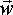# Physics - Kinematics - Angular Velocity ()

## Representing angular velocity using quaternions

If we let:

a(t) = a0 +w * t

where:

• a(t) = angle in radians that a solid body has turned through at a given time about a constant axis
• a0 = angle at time t=0.
• w = angular velocity in radians per second about a given axis.
• t = time

The quaternion representation of a rotation angle (orientation) is:

q = cos(angle/2) + i sin(angle/2)*axisx + j sin(angle/2)*axisy + k sin(angle/2)*axisz

where:

• axisx = component of axis in x dimension
• axisy = component of axis in y dimension
• axisz = component of axis in z dimension

so if the quaternion is a function of time we substitute angle for a0 +w * t to give:

q = cos(( a0 +w * t)/2) + i sin(( a0 +w * t)/2)*axisx + j sin(( a0 +w * t)/2)*axisy + k sin(( a0 +w * t)/2)*axisz

So this gives the orientation as a function of time, so to get angular velocity we need to differentiate this with respect to time, as discussed here, so we differentiate q to get qw which is a quaternion representing angular velocity.

So if we differentiate each term we get,

real terms ---> d(cos(( a0 +w * t)/2))/dt = d(cos(( a0 +w * t)/2))/d( a0 +w * t)/2) * d( a0 +w * t)/2)/dt = -sin( a0 +w * t)/2)*w

imaginary terms ---> d(sin(( a0 +w * t)/2))/dt = d(sin((a0 +w * t)/2))/d(( a0 +w * t)/2) * d(( a0 +w * t)/2)/dt = cos((a0 +w * t)/2)*w

So if we put the quaternion back together we get:

qw = dq/dt = w * (-sin(( a0 +w * t)/2) + i cos(( a0 +w * t)/2)*axisx + j cos(( a0 +w * t)/2)*axisy + k cos(( a0 +w * t)/2)*axisz)

So what is the physical interpretation of this? Unless I have made an error (which is very likely) an instantaneous rotation velocity can be represented by a quaternion but its elements would be continuously varying with time in a way that is difficult to interpret?

 metadata block see also: Correspondence about this page Open Forum - w x r or r x w ? Mati Mr. Dual Space Book Shop - Further reading. Where I can, I have put links to Amazon for books that are relevant to the subject, click on the appropriate country flag to get more details of the book or to buy it from them.Engineering Mechanics Vol 2: Dynamics - Gives theory for rigid dynamics, aims to allow prediction of effects of force and motion. Includes rotating frame of reference. Lots of colour diagrams, I guess its college / University level. Commercial Software Shop Where I can, I have put links to Amazon for commercial software, not directly related to the software project, but related to the subject being discussed, click on the appropriate country flag to get more details of the software or to buy it from them.Mathmatica

This site may have errors. Don't use for critical systems.